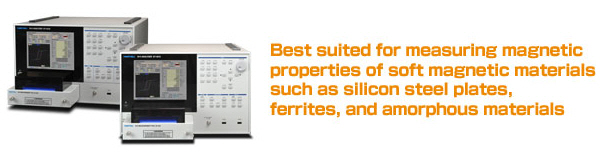Iwatsu B-H Analyzer SY-8219- Wide measurement frequency for materials analysis which used in high frequencies SY-8218: SINE from 10Hz to 10MHz, Pulse at Duty 50:50 from 10Hz to 1MHz SY-8219: SINE from 10Hz to 1MHz, Pulse at Duty 50:50 from 10Hz to 1MHz - 16times of acquisition data(comparing with current Iwatsu models) Acquisition data at 8,192points/cycle perform precise measurement on parameters such as Hc(Coersive force), Br(Residual fl ux density), and other parameters. - Pulse excitation function Both SINE(Sinusoidal) and Pulse(at Duty 50:50, 1MHz,max. ) excitation are available as a standard function - Temperature characteristic test with Scanner Chamber System - Optional Items Power Amplifiers DC bias power supply* Single plate measurement function* High-current POD*
SY-8218 SY-8219
Measurement method CROSS-POWER method (conformance to IEC62044-3)
Measurement mode
B-H measurement, Pc measurement, µ measurement
Measurement item

Characteristics value

 B-H measurement： Max. magnetic flux density (Bm), residual magnetic flux density(Br), max. magnetic field (Hm), coercive force (Hc), squareness ratio (Br/Bm), amplitude permeability (µa), core loss (Pc, Pcv, Pcm), current/voltage phase (θ), total magnetic flux change (2φm) Pc measurement： Max. magnetic flux density (Bm), residual magnetic flux density (Br), max. magnetic field (Hm), coercive force (Hc), amplitude permeability (µa), core loss (Pc, Pcv, Pcm), phase (θ), apparent power (VA) µ measurement： Max. magnetic flux density (Bm), max. magnetic field (Hm), impedance permeability (µz), complex permeability (µ, µ"), amplitude permeability (µa), core loss (Pc), phase (θ), loss coefficient (tanδ), inductance (L), resistance (R), impedance (Z), quality coefficient (Q)
Waveform display B-H curve, waveforms of excitation current, induced voltage, magnetic field, magnetic flux density
Measurement frequency 10 Hz to 10 MHz 10 Hz to 1 MHz
Magnetic field signal detection
Voltage drop at both edges of non-inductive resistor Maximum signal detection current: 6A
Magnetic flux density signal detection Voltage detection at both edges of induced voltage detection coil Maximum signal detection voltage:±200 V
Digitizer resolution 16 bits (8,192 points/cycle)
Sample connection method
2 or 1 coil (winding) method
Display Color LCD display (800 x 600 pixels)
Power supply
AC100 V to 240 V, 50/60 Hz, Power consumption: Approx. 130 VA MAX
Weight and dimensions Main unit: Approx. 12.5 kg, 420 (W) x 266 (H) x 480 (L) ±2 mm (without the projection section)
Interface
USB (data storage)